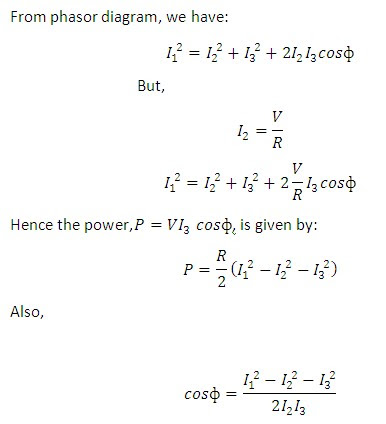# Three ammeter method

## Three ammeter method for measurement of power:

Following figures shows the circuit diagram and phasor diagram of three ammeter method for measurement of power. The current measured by the ammeter A1, is the vector sum of the load current and that taken by the non-inductive resistor R, this latter being in phase with V.

From phasor diagram, we have:• The advantage of this method is that the value of determined is independent of supply frequency and waveforms.

• The disadvantages of measurement of power by three voltmeter method are overcome in this method.

Let us solve one numerical based example on three ammeter method for clear understanding.

### Example 1:

The following readings were obtained from three ammeters used for a single phase power measurement: An inductive load takes a current of 2.5 A; a non-inductive resistor connected in parallel takes 2.4 A, when connected across 250 V supply. The total current taken from the supply is 4.5 A. Calculate:

a)    Power absorbed by the load.

c)     Power factor of the load.

### Solution:

Given: I3 = 2.5 A; I2 = 2.4 A; I1 = 4.5 A; V = 250 V.

Non-inductive resistance, R = (V/I2) = 250/2.4 = 104.17 O.

i)                  Power absorbed by the load, P:

P = (R/2)*(I1^2 - I2^2 - I3^2)

= (104.17/2)((4.5^2)-(2.4^2)-(2.5^2)) = 429.2 W     (Ans.)

Z = (V/I3) = (250/2.5) = 100 O   (Ans.)

iii)             Power factor of the load, cos ? = (I1^2 - I2^2 - I3^2) /2I2I3

= [(4.5^2)-(2.4^2)-(2.5^2)]/(2*2.4*2.5) = 0.687    (Ans.)

You may also like:

Three voltmeter method for measurement of power.